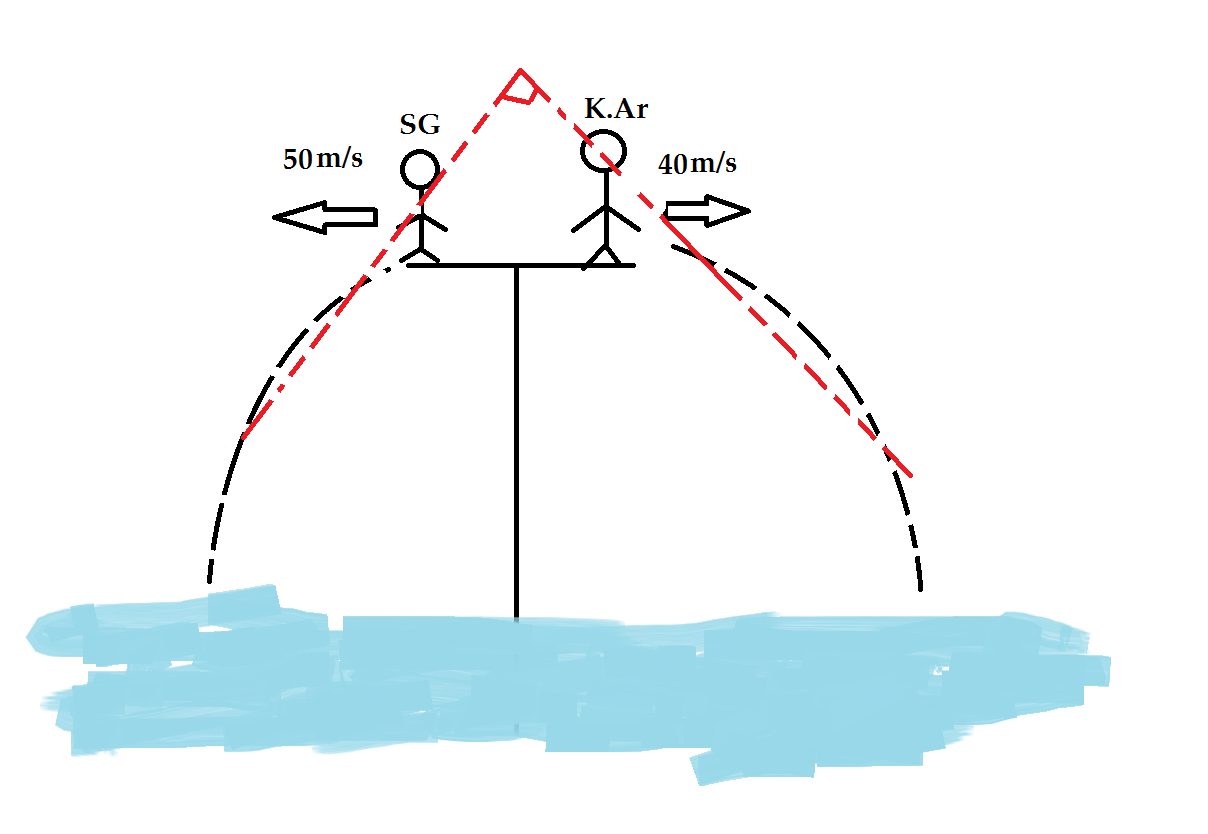On a hot summer evening, two Best Friends SG and Krishna decide to jump in a pool.

Both of them jumped into the pool at the same time and from the same spot. SG jumped with a horizontal velocity of $50 \ ms^{-1}$ and Krishna jumped with a horizontal velocity $40 \ ms^{-1}$ in the opposite direction.

The time at which their velocities are at $90^{\circ}$ with each other is of the form $\sqrt{m} \ s$. Find $m$.

(Take $g=10 \ ms^{-2}$. The image is not to scale.)

×